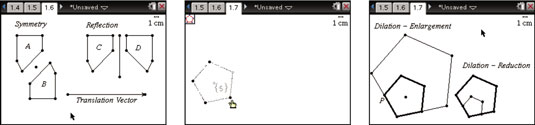##### TI-Nspire For DummiesThe Transformation menu provides five different transformations: Symmetry, Reflection, Translation, Rotation, and Dilation. Here’s a brief description of what four of the transformation tools can do and what you do to perform the transformation:

• Symmetry: This transformation gives the image of an object with a 180-degree rotation about a point.

• Reflection: This transformation gives the image of an object reflected over a line or segment.

• Translation: This transformation gives the image of an object translated by a distance and direction given by a vector or two points.

• Dilation: This transformation gives the image of an object with a point that is the center of dilation and a number specifying the dilation factor.

The first two transformations, symmetry and reflection, are accomplished using similar steps. Here are the steps to reflect a polygon over a line:

1. Draw a polygon.

2. Draw a segment or line.

3. Press [MENU]→Transformation→Reflection to open the Reflection tool.

4. Click the polygon.

5. Click the segment or line.

When using the Symmetry tool, click a point.

6. Press [ESC] to exit the Reflection tool.

Here are the steps for using the Translation tool:

1. Draw an object.

2. Draw a vector (or two points) that gives the direction and length of the translation.

3. Press [MENU]→Transformation→Translation to open the Translation tool.

4. Click the object.

5. Click the vector (or each of the two points).

6. Press [ESC] to exit the Translation tool.

The first screen shows the results of using the Symmetry, Reflection, and Translation tools. Start by drawing polygon A. Then use the Symmetry tool to complete a 180-degree rotation of polygon A about point p, which yields polygon B.The Translation tool was used to translate polygon A to polygon C. To complete this step, click polygon A and then the translation vector located at the bottom of the screen (to draw a vector, press [MENU]→Points & Lines→Vector). Notice that the corresponding points on polygons A and C are located at a distance and direction equal to the translation vector.

Finally, use the Reflection tool to complete the reflection of polygon C over the line. Try dragging objects and observing the corresponding changes.

To perform a dilation, follow these steps:

1. Draw a regular pentagon with the Regular Polygon tool. Press [MENU]→Shapes→Regular Polygon to open the tool.

2. Move the cursor to an existing point or any open space on the page and press [CLICK] to mark the starting point.

3. Use the Touchpad to move the cursor away from the center of the regular polygon and press [CLICK] to establish the radius and first vertex of the regular polygon.

4. Use the Touchpad to move the cursor in a clockwise direction. This action decreases the number of sides of the regular polygon. Press [ENTER] when the number of sides is 5.

Take a minute to play around with this tool. You can have up to 16 sides, and you can create stars and other shapes when you move to less than three sides.

In the classroom, you can use the Regular Polygon tool as a good visual to dynamically demonstrate that the more sides that you have, the closer the area of the polygon gets to the area of a circle with the same radius.

5. Press [MENU]→Transformation→Dilation to open the Dilation tool.

6. Click the point that defines the center of dilation.

7. Type a number to give the scale or dilation factor; then press [ENTER].

8. Click the object, which in this case is the pentagon.

9. Press [ESC] to exit the Dilation tool.

The third screen shows the result of using the Dilation tool. On the left is a dilation that results in an enlargement with a scale factor of 2 and center of dilation at point P. The image is shown with lines of medium weight, and the preimage is shown with lines of thin weight.

On the right is a dilation that results in a reduction with a scale factor of 2/3.